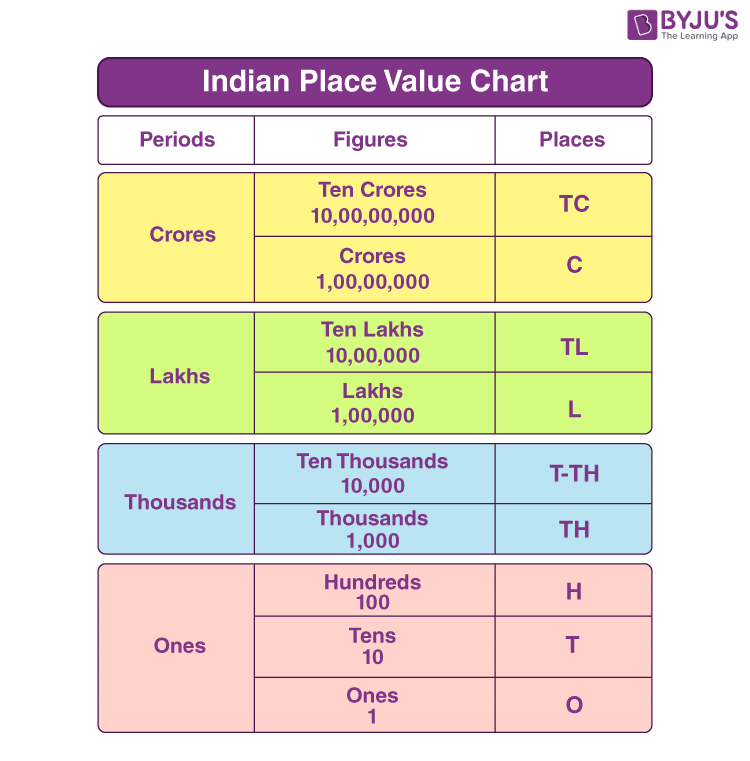# Indian Place Value Chart

The Indian place value chart will represent the place values of digits in a number. The position of a digit in a number is basically termed as place value. Now, to check if the digits are positioned correctly in a number, as per the Indian number system, we can use the place value chart. Also, with the help of this chart, we can learn to read and write the numbers in words. We will also discuss here the difference between the Indian place value chart and the international place value chart.

## What is the Indian Place Value Chart?

A chart that represents the place value of numbers as per the Indian number system is called the Indian place value chart. A number represents a count or a value. Each digit has its place value in the number, which tells its position. To learn the large numbers without any confusion, we use this chart to arrange them into periods. These periods are separated from each other using commas.

We usually go far up to nine places in the place value chart. The ninth place represents ten crores, and the first place shows the ones place. The order of place value is given from right to left for a whole number.

 Ten Crores One Crore Ten Lakhs One Lakh Ten Thousands Thousands Hundreds Tens Ones 10,00,00, 000 1,00,00,000 10,00,000 1,00,000 10,000 1,000 100 10 1

An example of a number is given below, using the Indian place value chart.

 Ten Crores One Crore Ten Lakhs One Lakh Ten Thousands Thousands Hundreds Tens Ones 1 2 2 0 4 5 9 1 2

The above number is 12, 20, 45, 912. If we spell it in words, the number would be Twelve crores, twenty-lakhs, forty-five thousand, nine hundred twelve.

## Indian Place Value Chart – Periods

If we draw the chart for the Indian place value system based on period, then follow the below rules:

• First Period: In the above chart, the first period will include three-place values, i.e., ones, tens and hundreds.
• Second Period: The second period in the chart includes two place values, i.e., thousands and ten thousands.
• Third Period: In the Indian place value chart, the third period will include two places for lakhs and ten lakhs.
• Fourth Period: The fourth period will have two places, for crore and ten crores.## Indian Place Value Chart for Decimal

For decimal numbers, the place value is defined for digits from left to the right after the decimal point.

 Tens Ones Decimal point Tenths Hundredths Thousands 10 1 . 1 2 3

## Difference Between Indian and International Place Value Chart

The lakhs and crores in the Indian system are not represented the same as the international number system. This is the main difference between them, which is expressed through putting commas differently in a number. Let us find more differences in the below table.

 Indian Place Value Chart International Place Value Chart The order of place values of digits are: Ones, Tens, Hundreds, Thousands, Ten thousands, Lakhs, Ten lakhs, Crores, Ten crores. The order of place values of digits are: Ones, tens, hundreds, thousands, ten thousands, hundred thousands, millions, ten million, and hundred million. The nine places in the chart are grouped into four periods. The nine places in the chart are grouped into three periods. The digits are separated by commas in the form of 3,2,2, starting from right to left in a number. The digits are separated by commas in the form of 3,3,3, starting from right to left in a number. Example: 1, 32, 24, 54, 900 Example: 1, 322, 454, 900

## Solved Examples on Indian Place Value Chart

Q.1: Express the number 92357385 in a place value chart.

Solution: The given number, 92357385, has eight digits.

As per the Indian number system, we can write the number as: 9, 23, 57, 385

 One Crore Ten Lakhs One Lakh Ten Thousands Thousands Hundreds Tens Ones 9 2 3 5 7 3 8 5

Q.2: Express the number 90982.781 in an Indian place value table.

Solution: Given the number is 90982.781. Since it is a decimal value, we have to add the place values for the digits after the decimal point.

 Ten thousands Thousands Hundreds Tens Ones Decimal point Tenths Hundredths Thousands 9 0 9 8 2 . 7 8 1

Thus, it can also be written as 90, 982.781.

## Frequently Asked Questions on Indian Place Value Chart

Q1

### What is the chart of Indian Place Value?

Indian place value chart is a table that is used to represent the positions of digits in a number. It is most commonly used for expressing large numbers, such as in lakhs or in crores. The order of position of digits as per the Indian number system is ones, tens, hundreds, thousands, ten thousands, lakh, ten lakhs, crore, ten crores, and so on.

Q2

### How does the Indian place value chart differ from that of the International one?

The order of place value of digits is different for both the number systems. The chart basically holds for 9 places for which the Indian place value chart will have four periods, while the international place value chart will have three periods.

Q3

### What comes after crore in the Indian place value system?

After crores, ten crores come in the Indian place value chart, at 9th place.